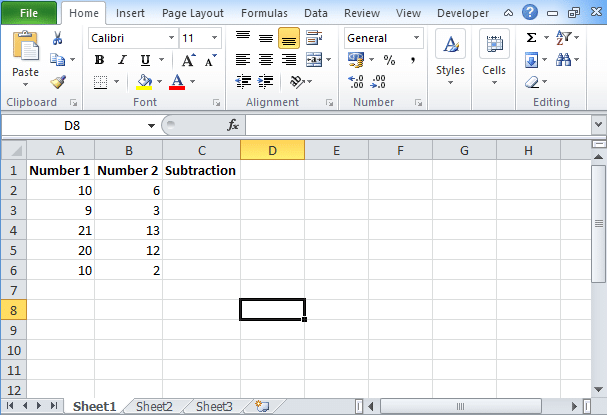To advertise with us contact on Whatsapp: +923041280395 For guest post email at: [email protected]

# Excel Subtraction Formula: How to use Subtract FunctionThere are various excel formulas basic including excel subtraction formula and advanced available in Microsoft Excel that you can use for different purposes. You might have used sum and average functions in excel as its icon is available at Excel ribbon. But you will not find an icon for subtraction. So if you want to minus one value from another value then you need to use excel subtraction formula. Check the process to apply this formula and subtract one value from other value.

## Excel Subtraction Formula

In the excel subtraction formula, you have to start it from “= sign”. With the = sign, you need to put two or more numbers with “-“operator in between these numbers. For example, if you want to subtract 3 and 6 from 13, then you need to write the formula as =13-3-6. This will give you result as 4. To perform subtraction on given numbers, you need to put formula. Before embedding this formula, you need to focus on these points.

• The excel formula will begin with an equal sign “=”.
• You need to type this formula where you want that your subtracted value appears.
• You need to take the sign (-) as the subtraction sign.
• Press the enter key to complete the formula.

## How to use Excel Subtraction Formula?

Similarly like addition, subtraction of numbers together is very easy. All you need to apply is the subtraction formula using subtraction operator. Check the steps:

• Let’s take a table, in column A we have taken Minuend and in column B we will take subtrahend.
• Enter the numbers in A column and in column B.
• In column C, you have to enter the subtraction formula
• Subtraction formula is =A1-B1.
• Drag right corner of the cell to downwards on rest of the cell.
• You will find the subtracted outcome value in the result column.

## Change the data

To examine the subtraction formula, make changes in cell A or B and then press enter. You will find that the answer in column C will automatically get changed. Not only subtraction, but you can also perform other excel functions such as division and addition.

## Types of subtraction performed

Subtraction between numbers is performed in a single cell or within the range of cells. You can perform subtraction in different ways:

### Subtraction between simple numbers

For this, you can use a single cell on which you can perform subtraction problems. This is quite simple, but you can apply this subtraction formula on large numbers and too complex data.

### Subtraction between 2 or more cells

Every cell has its own cell reference that denotes the column and row number. To execute subtraction, you have to use cell references. For example, if you want to subtract the value available in cell A with cell B then your subtraction formula will be =B1-A1.

## Conclusion

Depending on the data type, you can perform a subtraction between numbers quickly and in a simple way. There are several methods to apply to excel subtraction formula between two numbers. Similarly, you can also perform other mathematical functions.